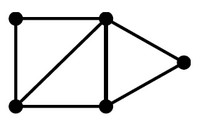# Graph - Operator (Operations)

## List

Operations Description
degree(G, x) the number of adjacent (connected) edge for the node x (ie degree)
adjacent(G, x, y) adjacent tests whether there is an edge from the vertex x to the vertex y;
neighbors(G, x) lists all vertices y such that there is an edge from the vertex x to the vertex y;
remove_vertex(G, x) removes the vertex x, if it is there;
add_edge(G, x, y) adds the edge from the vertex x to the vertex y, if it is not there;
remove_edge(G, x, y) removes the edge from the vertex x to the vertex y, if it is there;
get_vertex_value(G, x) returns the value associated with the vertex x;
set_vertex_value(G, x, v) sets the value associated with the vertex x to v.
get_edge_value(G, x, y) returns the value associated with the edge (x, y);
set_edge_value(G, x, y, v) sets the value associated with the edge (x, y) to v.

## Documentation / Reference

Discover More